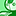# New to Qlik Sense

If you’re new to Qlik Sense, start with this Discussion Board and get up-to-speed quickly.

Announcements
Skip the ticket, Chat with Qlik Support instead for instant assistance.
cancel
Showing results for
Did you mean:Creator II

## Column total incorrect in a table (QlikSense)

Hi all,

I'm calculating a few measures using the following formulas.

Total Sales = sum(aggr(price, id, buyer))

Total Cost= sum(aggr(cost, id))

Reported Profit Margin= (Total Sales- Total Cost)/Total Sales

Now, a few buyers have a "adjusted profit margin" value and rest have null. Using this field I'm calculating New Sales as:

New Sales= Total Sales / ((1+ Reported Profit Margin) * (1 + Adjusted Profit Margin)) =

when I calculate the new sales measure, the individual New Sales values of the buyers are correct but the column total ( of New Sales)  is same as the Total Sales. I'm confused  about why this is happening.

I appreciate any help with this. Please let me know if I need to modify anything or if there is any better way to do this.

Thanks.

1 Solution

Accepted SolutionsMVP

Wrap you expression around with Sum(Aggr(Expression, Dimension/s)) which will fix your total....

2 RepliesMVP

Wrap you expression around with Sum(Aggr(Expression, Dimension/s)) which will fix your total....Creator II
Author

Thanks, SunnyTags
Community Browser